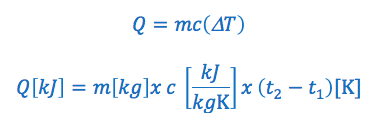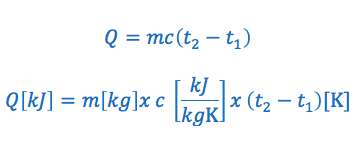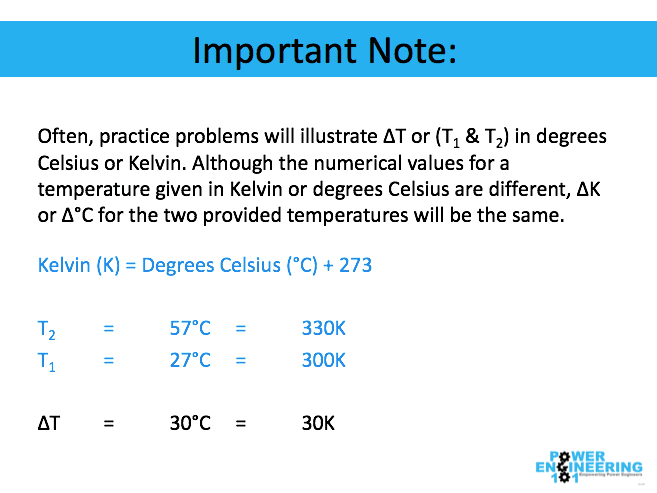# How to Solve Specific Heat Capacity Problems (3rd Class)

Todays tutorial covers How to Solve Specific Heat Capacity Problems to prepare you for your Third Class 3A1 exam.

The tutorial explains the concept of specific heat capacity and includes a Free PDF Download with several examples of how to use the formula, find the specific heat and how to use specific heat in calculations to solve specific heat capacity problems.

### What is Specific Heat Capacity? (Commonly Referred to as Specific Heat)

Specific heat of a substance is defined as the amount of heat required to raise the temperature of one kilogram of substance by one degree Kelvin (or one degree Celsius).

Note: Specific heat capacity of a substance is commonly referred to as simply the specific heat of the substance.

### Specific Heat Capacity Formula:

From the above definition of specific heat we can conclude that the quantity of heat energy transferred to the substance is dependent upon the product of the following:

• The mass of the substance.
• The specific heat of the substance.
• The temperature change of the substance.

The specific heat capacity formula is commonly written in the following two ways:OR### Specific Heat Capacity Formula Variables:

Q = is the quantity of heat added or removed. If it is greater then zero heat is added, if it is less then zero heat has been removed. The quantity of heat added or removed is typically expressed in kilojoules “kJ”

m = is the mass of the substance. Typically expressed in kilograms “kg”

c = is the specific heat of the substance. Typically expressed in kilojoules per kilogram per kelvin “kJ/kGK” or kilojoules per kilogram per °C “kj/kg°C”

ΔT = is the change in temperature. Typically in Kelvin or degrees Celsius for temperature.

T1 = The initial temperature of the substance. Typically in Kelvin or degrees Celsius for temperature.

T2 = The final temperature of the substance. Typically in Kelvin or degrees Celsius for temperature.

Important note: Commonly practice problems will illustrate ΔT or (T1 & T2) in degrees Celsius or Kelvin. Although the numerical values for a temperature given in Kelvin or degrees Celsius are different, ΔK or Δ°C for the two given temperatures will be the same. For example:### How to Solve Specific Heat Capacity Problems Summary:

Let me know your thoughts of this new format in the comments below!